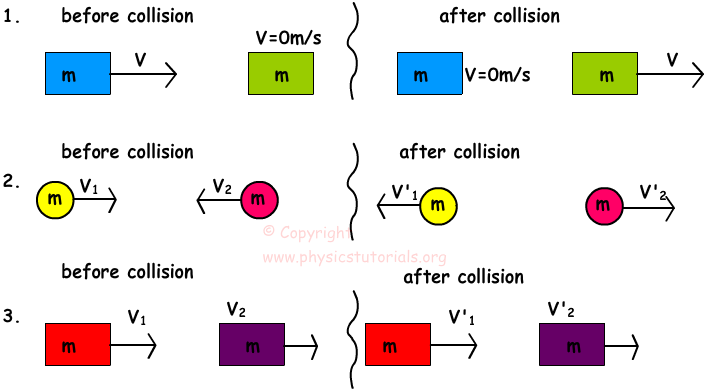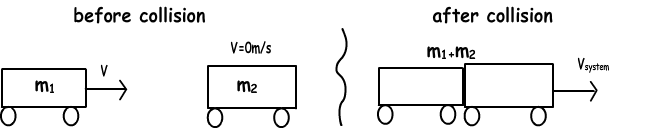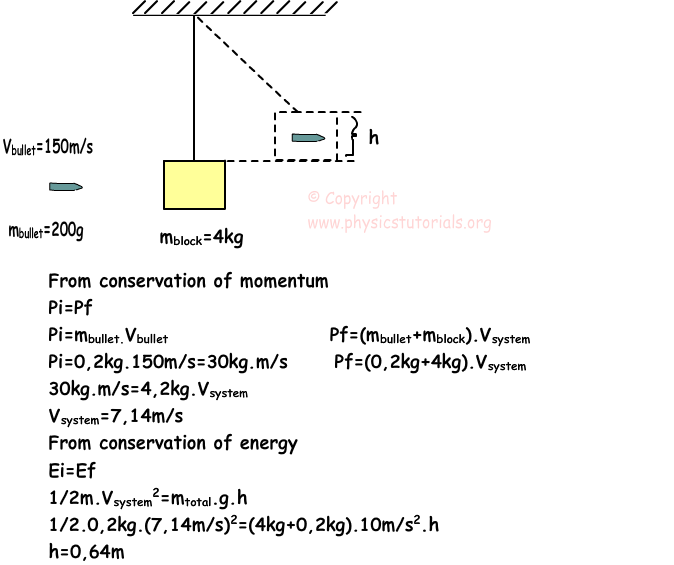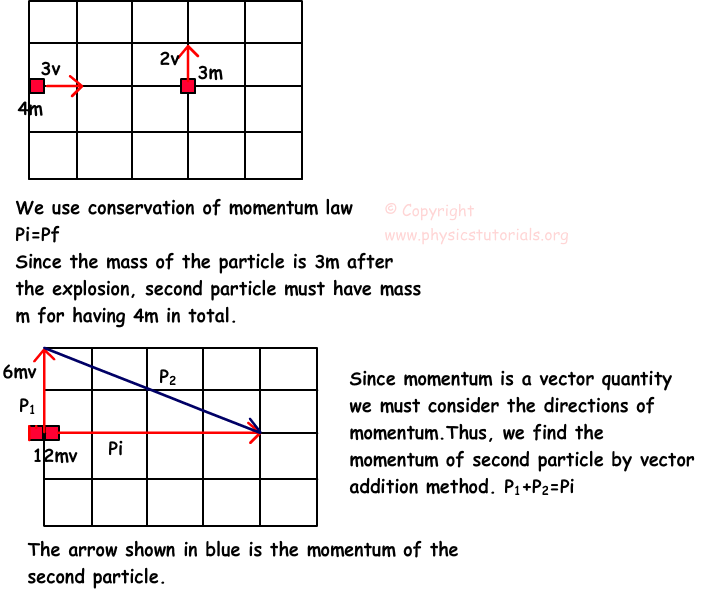## Collisions

COLLISIONS

Momentum is conserved in all collisions. However, we can examine collisions under two titles if we consider conservation of energy. For example, if the objects collide and momentum and kinetic energy of the objects are conserved than we call this collision “elastic collision”. On the other hand if the momentum of the object is conserved but kinetic energy is not conserved than we call this type of collision “inelastic collision”. In in elastic collisions some of the kinetic energy is converted to heat and sound.

The picture given below shows the examples of elastic collision in which both kinetic energy and momentum of the system are conserved.In this picture, which is an example of inelastic collision, momentum of the objects is conserved however; kinetic energy of the objects is not conserved.To sum up, we can say that, momentum of the system is conserved in both elastic and inelastic collisions however; kinetic energy is conserved only in the elastic collisions.

Example: A bullet which has velocity 150m/s and mass 4kg sticks to the stationary block. They move together after the collision. Find the height they reach after the collision.Example: Look at the given picture below. Particle having mass 4m and velocity 3v explodes and breaks into two pieces. One of the pieces has mass 3m and velocity 2v. Find the second momentum of the second particle and show it in a given diagram.Author: